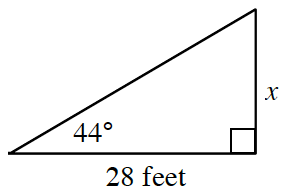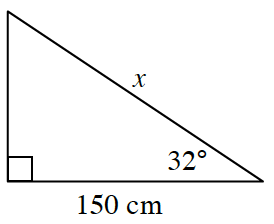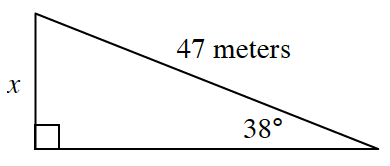### Home > INT3 > Chapter 6 > Lesson 6.2.2 > Problem6-46

6-46.

In each of the following triangles, what is the length of the side labeled $x$?

Use sine, cosine, and/or tangent to solve for the missing side lengths.

1.Use the tangent ratio.

1.$\approx176.88 \text{ cm}$

1.$\approx 28.94 \text{ meters}$